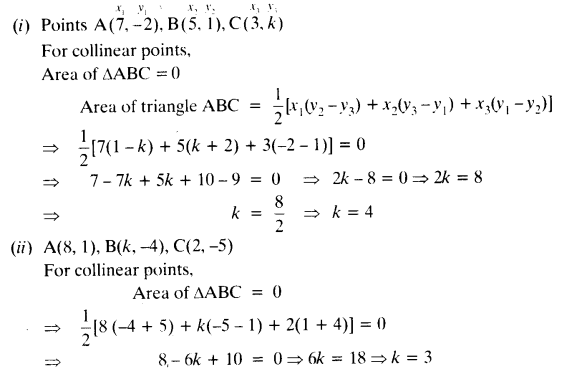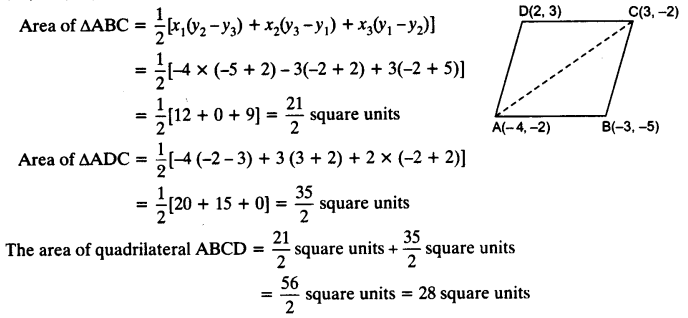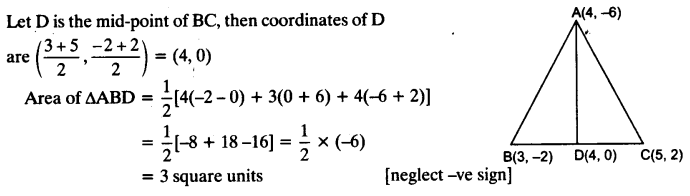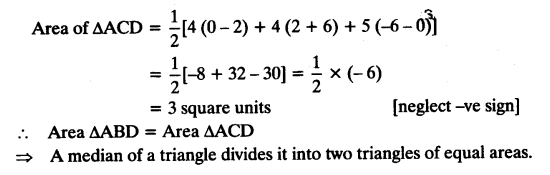NCERT Solutions for Class 10 Maths Chapter 7 Coordinate Geometry Ex 7.3 are part of NCERT Solutions for Class 10 Maths. Here we have given NCERT Solutions for Class 10 Maths Chapter 7 Coordinate Geometry Ex 7.3.

 Board CBSE Textbook NCERT Class Class 10 Subject Maths Chapter Chapter 7 Chapter Name Coordinate Geometry Exercise Ex 7.3 Number of Questions Solved 5 Category NCERT Solutions

## NCERT Solutions for Class 10 Maths Chapter 7 Coordinate Geometry Ex 7.3

Question 1.
Find the area of the triangle whose vertices are:
(i) (2, 3), (-1, 0), (2, -4)
(ii) (-5, -1), (3, -5), (5, 2)
Solution:Question 2.
In each of the following find the value of ‘k’ for which the points are collinear.
(i) (7, -2), (5, 1), (3, k)
(ii) (8, 1), (k, -4), (2, -5)
Solution:Question 3.
Find the area of the triangle formed by joining the mid-points of the sides of the triangle whose vertices are (0, -1), (2, 1) and (0, 3). Find the ratio of this area to the area of the given triangle.
Solution:Question 4.
Find the area of the quadrilateral whose vertices, taken in order, are (-4, -2), (-3, -5), (3, -2) and (2, 3).
Solution:Question 5.
You have studied in Class IX, that a median of a triangle divides it into two triangles of equal areas. Verify this result for ∆ABC whose vertices are A (4, -6), B (3, -2) and C (5, 2).
Solution:We hope the NCERT Solutions for Class 10 Maths Chapter 7 Coordinate Geometry Ex 7.3, help you. If you have any query regarding NCERT Solutions for Class 10 Maths Chapter 7 Coordinate Geometry Ex 7.3, drop a comment below and we will get back to you at the earliest.